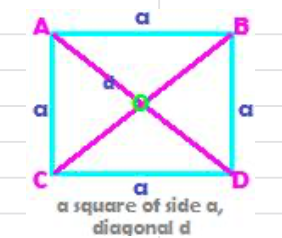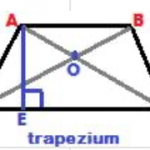Perimeter and area formulas for various quadrilateral shapes are given below:

Perimeter is sum of the lengths of the enclosing sides of a figure.

Area is the amount of plane region enclosed by the sides of the figure.

## 1. Perimeter and area of a parallelogram

In a parallelogram, opposite sides are equal in length. Therefore,

Perimeter of the parallelogram ABCD = AB + BC + CD + AD = 2AB + 2BC

Area of a parallelogram = base × height = b × h

## 2. Perimeter and area of a rhombus

In a rhombus, all four sides are equal in length. Therefore,

Perimeter of a rhombus ABCD = 4a, where a is length of each side

Area of a rhombus = ½ × (product of diagonals)

## 3. Perimeter and area of a rectangle

In a rectangle, opposite sides are equal in length.

One side is called length, usually the longer, and the other is called breadth, the shorter side.

If l denotes length and b, the breadth, then

Perimeter of a rectangle = 2(l + b), and

Area of rectangle = l × b

##In a square, all four sides are equal in length.

If each side is of length a, then

Perimeter of square = 4a, and

Area of square = a2

##In a trapezoid, one pair of sides is parallel to each other. The other two sides may be equal in length, in which case the quadrilateral is called isosceles trapezoid, or else they may be unequal in length.

Perimeter of a trapezoid = sum of the four sides.

In the trapezoid, AE is the distance between the parallel sides AB and CD, So,

Area of a trapezoid =
½ × (sum of the parallel sides) × (distance between the parallel sides)